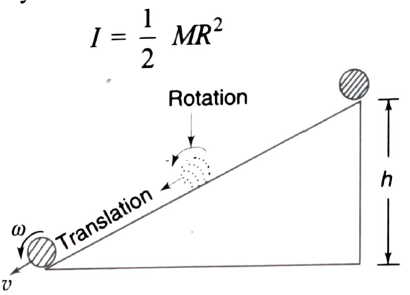Rolling motion
Question

# A solid cylinder of mass M and radius R rolls down an inclined plane of height h. The angular velocity of the cylinder when it reaches the bottom of the plane will be

Moderate
Solution

## Let M be the mass of the cylinder and R be its radius. When it is at the top of the inclined plane of height h, its potential energy is Mgh (Figure). As it rolls down the inclined plane, it moves along the plane and also rotates about an axis passing through its axis perpendicular to the plane of the figure, which shows its section in the plane of the paper. It thus acquires both kinetic energy of translation $\left(\frac{1}{2}{\mathrm{Mv}}^{2}\right)$ and kinetic energy of rotation $\left(\frac{1}{2}{\mathrm{I\omega }}^{2}\right)$ where v is its linear velocity and $\mathrm{\omega }$ its angular velocity when it reaches the bottom of the plane. I is its moment of inertia about the axis mentioned above which is given byFrom the law of conservation of energy, we havePotential energy = Translational kinetic energy + Rotational kinetic energy

Get Instant Solutions